> 文档中心 > AHP层次分析法与python代码讲解（处理论文、建模）

# AHP层次分析法与python代码讲解（处理论文、建模）

AHP是啥

①构建阶梯层次结构

②构建判断矩阵

④综合算术平均法 、几何平均法、特征值法求权重

③对判断矩阵进行一致性检验

⑤填充矩阵，得出结果

AHP扩展知识

# AHP是啥

层次分析法（analytic hierarchy process）简称AHP。是建模比赛中比较基础的模型之一，其主要解决评价类的问题，毕业论文中也经常能见到，可用于绩效评价。如选择哪种方案最好，哪位员工表现最好等。

该方法本质就是把一个决策问题根据评价的标准将其分为多层次结构，这些层次按照一定的关联关系构成自上而下的一种阶梯构造，再根据最底下的层次到高层次通过两两进行对比得出各个因素的权重，权重最大的就是这些方案中最好的方案或因素。

本文借鉴了数学建模清风老师的课件与思路，如果大家发现文章中有不正确的地方，欢迎指正，也可以点击链接查看清风老师视频讲解：清风数学建模：https://www.bilibili.com/video/BV1DW411s7wi

AHP 的主要计算可分为五个过程

# 题目

小明同学想出去旅游。在查阅了网上的攻略后，他初步选择了苏杭北戴河桂林三地之一作为目标景点。请你确定评价指标、形成评价体系来为小明同学选择最佳的方案。

①我们评价的目标是什么？

答：为小明同学选择最佳的旅游景点。
②评价的准则或者说指标是什么？（我们根据什么东西来评价好坏）

题目没给相关数据，需要我们确定。

（一般而言，①③这两个问题的答案是显而易见的，问题②的答案需要我们根据题目中的背景材料、常识以及网上搜寻到的参考资料进行结合，从中筛选出最合适的指标）

答：这里我们选择了景色、花费、居住、饮食、交通。
③我们为了达到这个目标有哪几种可选的方案 ？

答：三种，分别是去苏杭、去北戴河和去桂林。

# ①构建阶梯层次结构• 其中，蓝色一列代表景色、花费、居住、饮食以及交通的权重，加和为1（有时候加起来不是1是因为四舍五入的原因）。（实际上就是准则层关于上一层目标层的重要性）
• 然后同一颜色每一横行，就是三种方案相对于准则层的重要性。

# ②构建判断矩阵

建立阶梯层次结构模型时，有必要清楚模型中各个因素之间的联系， 这中联系在 AHP 中要用矩阵法来表现出来，就是对各个指标之间采用 两两比较 ， 用 cij 表示元素 i 对元素 j 的注重程度比较值，以数字从 1 9 还有它的倒数作为比较标准，然后对评价指标进行打分排列，判断矩阵就是在这个基础上建立得出的。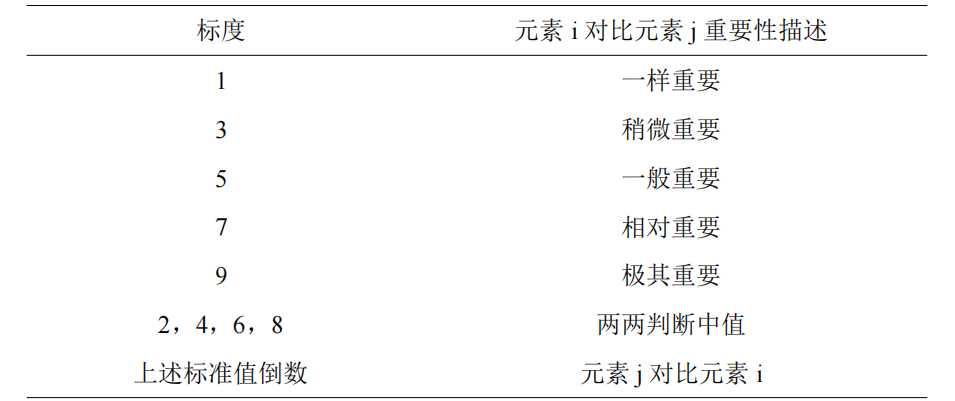矩阵个数确定 ：由上文所讲内容可知得到这个判断矩阵实际上要分别得出准则层关于目标层的一组权重向量，方案层关于准则层的五组权重向量，实际上我们就需要构造出一个准则层关于目标层的判断矩阵以及五个方案层关于准则层的矩阵，一共六个判断矩阵（如下）。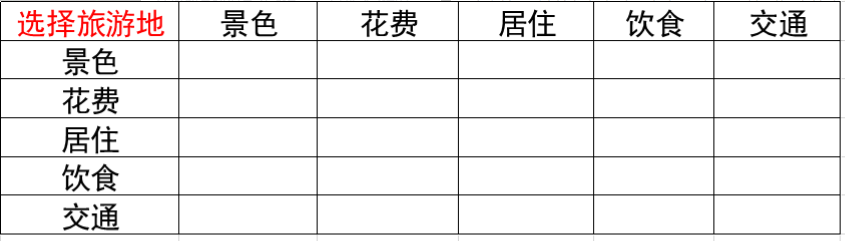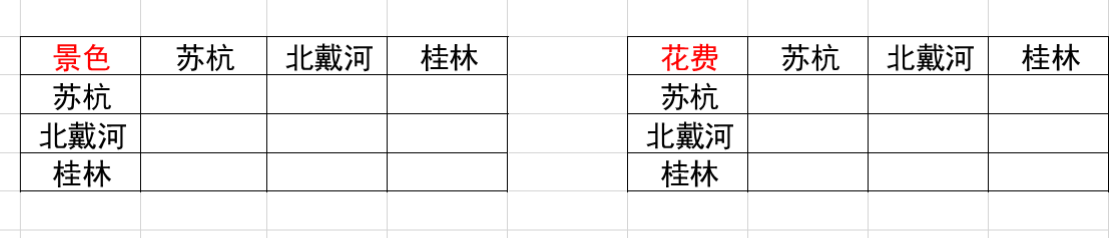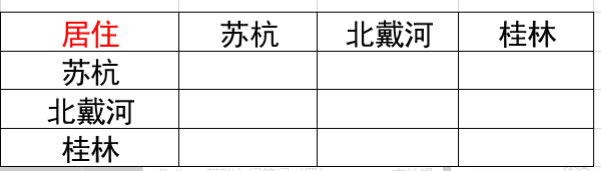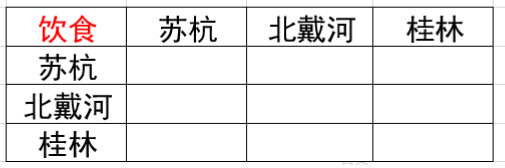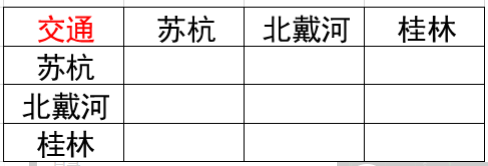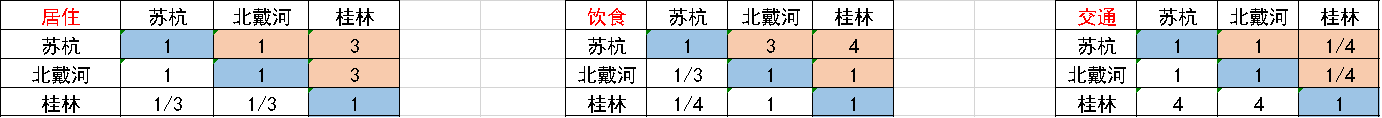①aij 表示的意义是，与指标j相比， i的重要程度。（注意i是行j是列，笔者经常搞混）

②主对角线元素为1原因：当i= j时，两个指标相同，因此同等重要记为1

③aj >0 且满足aij = 1/aji，也就是 aij × aji = 1 (我们称满足这一条件的矩阵为正互反矩阵)

# ④综合算术平均法 、几何平均法、特征值法求权重

①算数平均法求权重

②几何平均法求权重## 方法1：算术平均法求权重

### 一般步骤### 表达式解释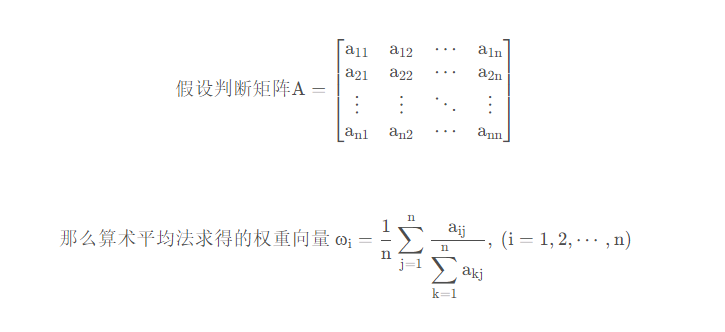### 代码实现

``import numpy as npfrom numpy import linalg# np.set_printoptions(precision=4)'''算术平均法权重'''def arithmetic_mean(a):    n = len(a)    b = sum(a)    print('b:', b)    # 归一化处理    normal_a = a/b    print("算术平均法权重-归一化处理：")    print(normal_a)    average_weight = []    for i in range(n): s = sum(normal_a[i]) print("第{}行求和 ".format(i+1), s) # 平均权重 average_weight.append(s/n)    # print(average_weight)    print('算术平均法权重:')    print(np.array(average_weight))    return np.array(average_weight)``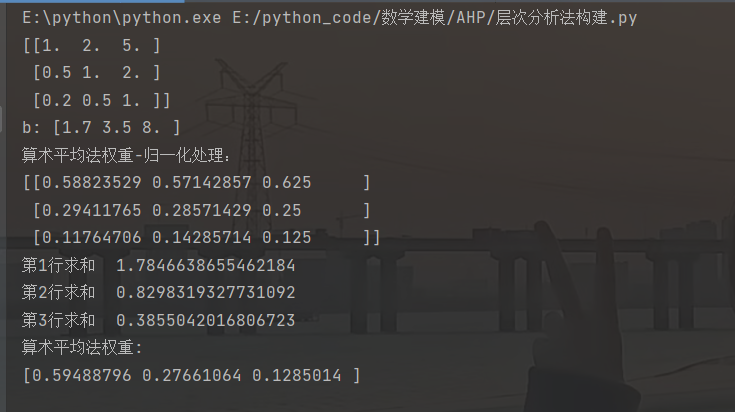①如果你想全篇设置四位小数点，在开头加上这个

``np.set_printoptions(precision=4)``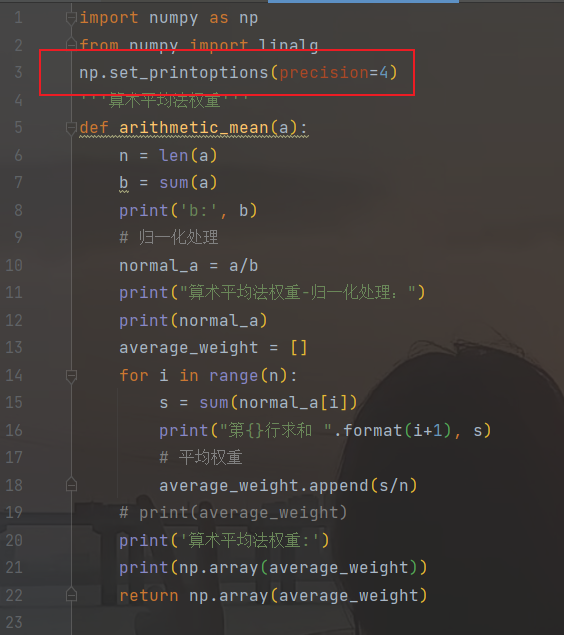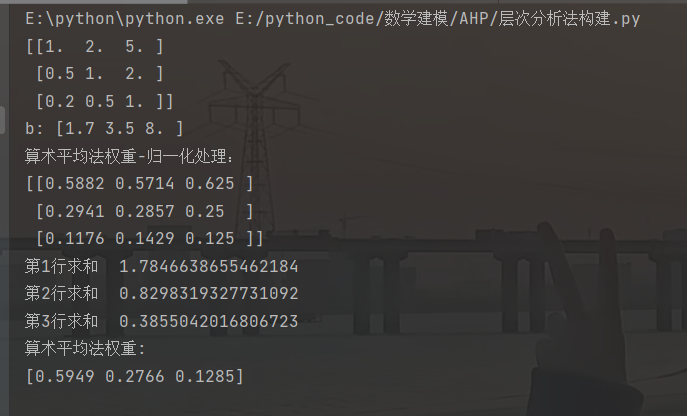②对二维数组使用sum函数，最终形成的是一个包含每列的和的数组。

③代码for循环中进行了上述步骤二和三，先对每行求和，然后除以n，得到了权重。

## 方法2：几何平均法求权重

### 表达式解释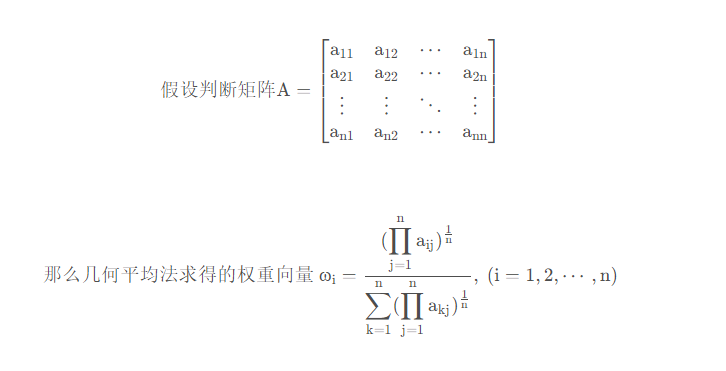### 代码实现

``'''几何平均法求权重'''def geometric_mean(a):    n = len(a)    # 1表示按照行相乘，得到一个新的列向量    b = np.prod(a, 1)    print(b)    c = pow(b, 1/n)    print(c)    # 归一化处理    average_weight = c/sum(c)    print('几何平均法权重:')    print(average_weight)    return average_weight``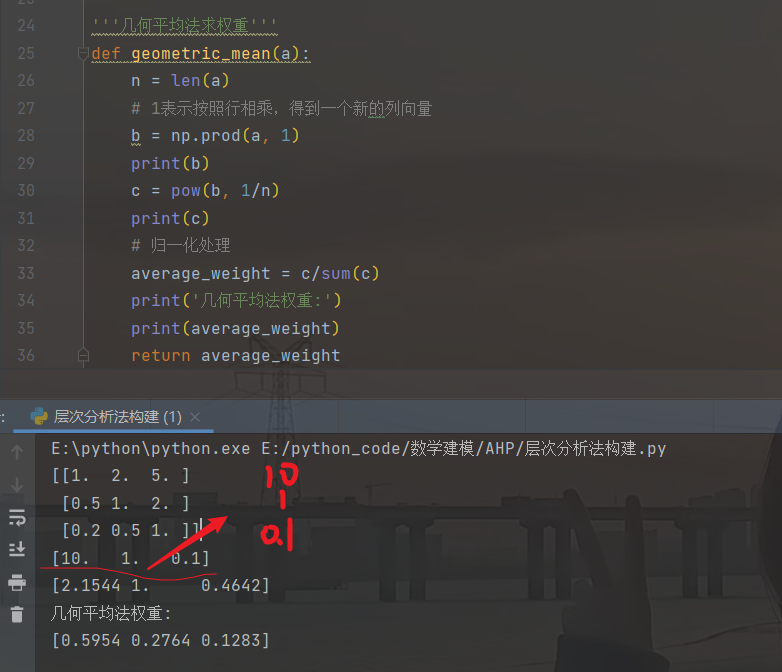c为开n次方的数组。## 方法3：特征值法求权重

在了解 这个之前，你得大致了解一下什么是特征值，特征向量，最大特征值（上文建议好好看看前半部分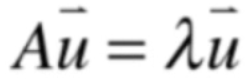### 代码实现

``'''特征值法求权重'''def eigenvalue(a):    w, v = np.linalg.eig(a)    for i in range(len(w)): print('特征值', a[i], '特征向量', v[:, i])    index = np.argmax(w)    w_max = np.real(w[index])    vector = v[:, index]    vector_final = np.transpose(np.real(vector))    print('最大特征值', w_max, '对应特征向量', vector_final)    normalized_weight = vector_final/sum(vector_final)    print('***归一化处理后:', normalized_weight)    return w_max, normalized_weight``

np.linalg.eig() 就是用来计算正方形数组的特征值与特征向量的 。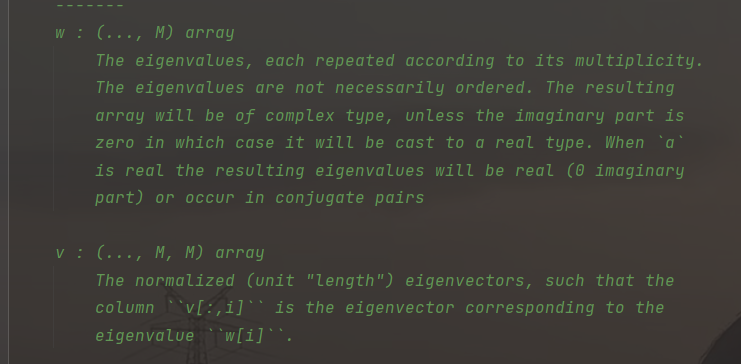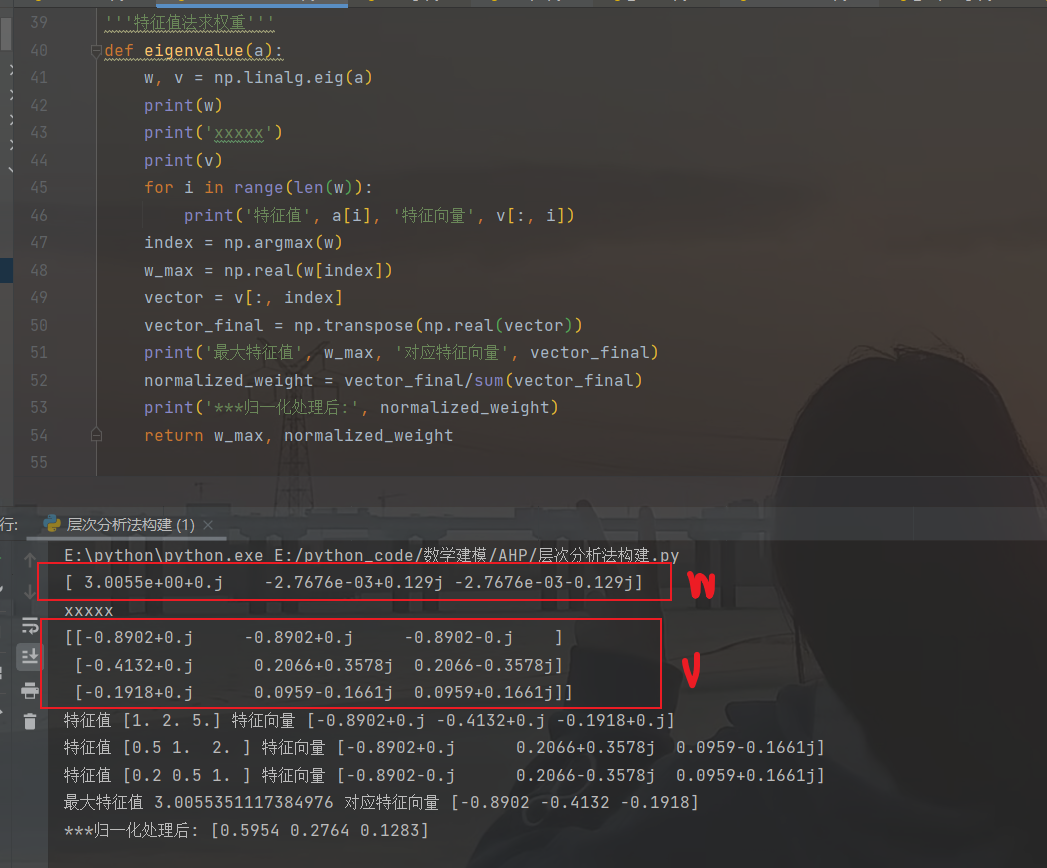``np.argmax(w)``

``np.real()``

``vector = v[:, index]``

`vector = v[:, 0]表示取数组的第0列`
`vector = v[:, 1]表示取数组的第1列`
`vector = v[0，:]表示取数组的第0行`
`vector = v[1，:]表示取数组的第1行`

``w_max = round(np.real(w[index]),4)``

## 求综合平均权重

``'''综合平均权重'''def average_Weight(a):    am = arithmetic_mean(a)    gm = geometric_mean(a)    ev = eigenvalue(a)    aw = np.array([am, gm, ev])    print(aw)    final_weight = sum(aw)/3    print(final_weight)    return final_weight``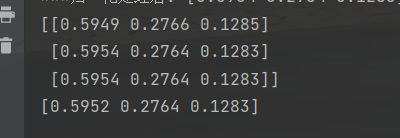# ③对判断矩阵进行一致性检验

（aj >0 且满足 aij × aji = 1)

``'''判断正互反矩阵'''def reciprocal_matrix_judge(a):    print(a)    n = len(a)    b = 0    for j in range(n): for i in range(n):     if a[:, j][i] * a[j, :][i] == 1:  b += 1    if b == n*n: print("该矩阵是正互反矩阵！\n") return True    else: print("该矩阵不是正互反矩阵！\n") return False``

这里笔者使用自己的理解写的python判断正互反矩阵的代码（网上貌似没找到有人写过），由于笔者对数组这些不太熟，就不是按照定义上判断aij是否等于1/aji而是采用判断aij与aji乘积为1的个数，如果刚好为数组元素的个数，也是数组行数或者列数的平方（因为两两比较，所以是个方阵）

当然，期待有人使用定义或者其他更好的方法进行判断正互反矩阵。①     aij = i的重要程度 / j的重要程度，

ajk = j的重要程度 / k的重要程度，

aik = i的重要程度 / k的重要程度 = aij × ajk。
②    矩阵各行（各列）之间成倍数关系。
我们进行构造矩阵时难免会出现矛盾，即不容易构造出一致性矩阵，但是我们可以向一致性矩阵靠拢，只要这个差距（CR）不超过一个范围（0.1）那么这个判断矩阵也是可以使用的。这个判断差距的过程叫做一致性检验。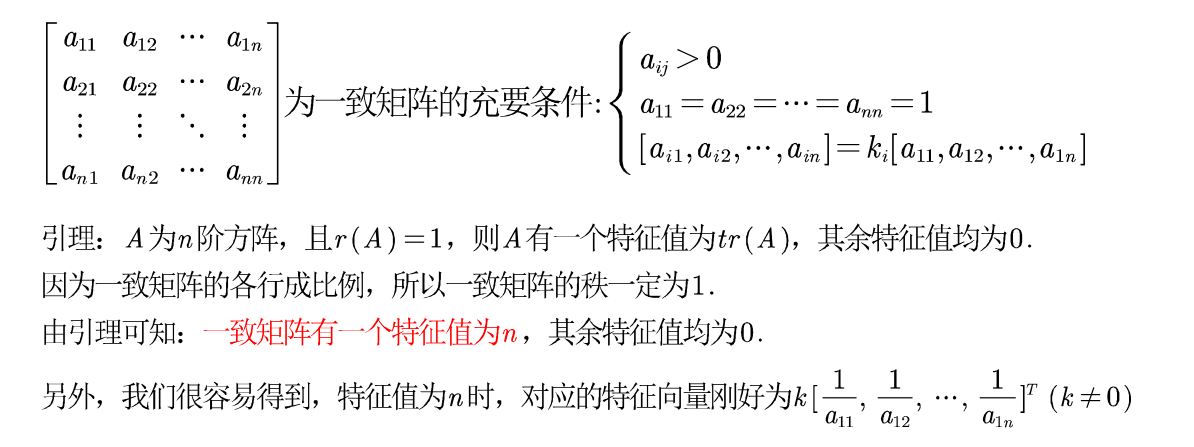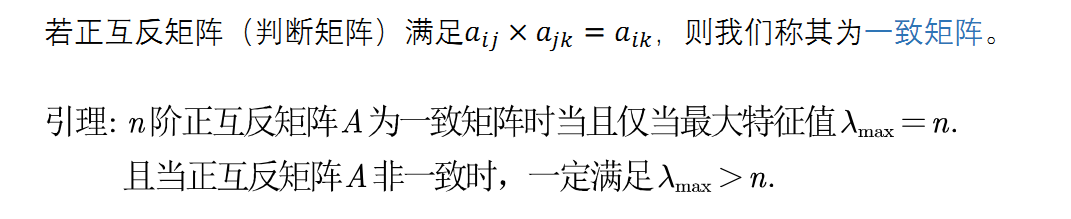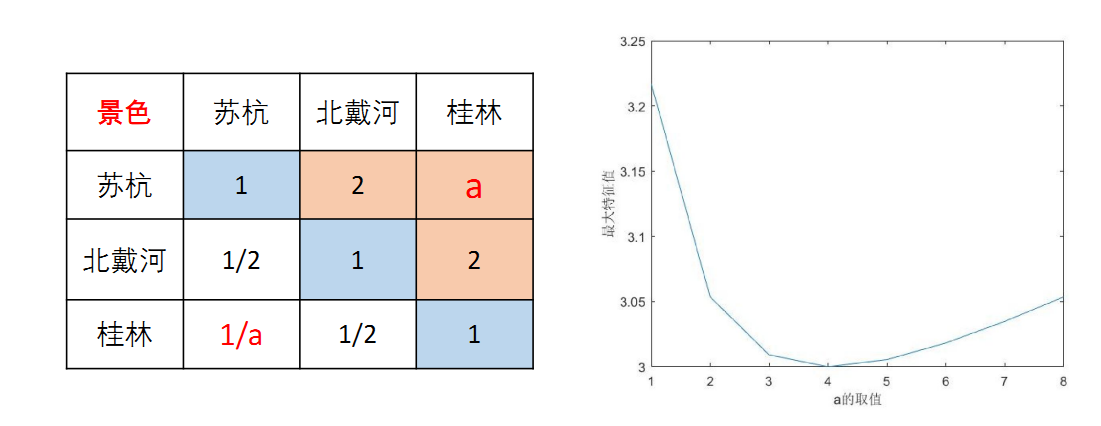## 一致性指标CI计算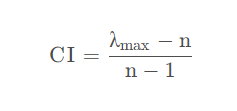``'''CI计算'''def CI_calc(a):    n = len(a)    λ_max = eigenvalue(a)    print(λ_max)    CI = (λ_max-n)/(n-1)    print('CI:', CI)    return CI``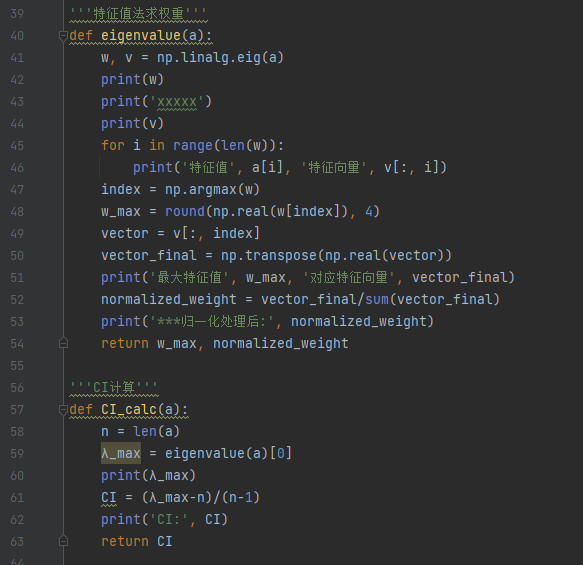eigenvalue()是上文提到的特征值法求权重，由于我们需要 λ_max（最大特征值），其是eigenvalue()中第一个返回的w_max。然后使用CI的计算公式计算即可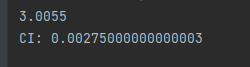## 一致性比例CR计算``'''CR计算'''def CR_calc(a):    RI = np.array([0, 0, 0.52, 0.89, 1.12, 1.26, 1.36, 1.41,     1.46, 1.49, 1.52, 1.54, 1.56, 1.58, 1.59])    n = len(a)    CI = CI_calc(a)    CR = CI/RI[n-1]    print('CR:', CR)    if CR < 0.1: print("一致性检验通过！\n") return True    else: print("一致性检验失败，请修改！") return False``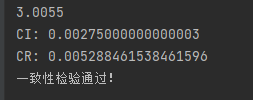``if __name__ == '__main__':    a = np.array([[1, 2, 5],[1 / 2, 1, 2],[1 / 5, 1 / 2, 1]])    rmj = reciprocal_matrix_judge(a)    if rmj: if CR_calc(a):     average_Weight(a)``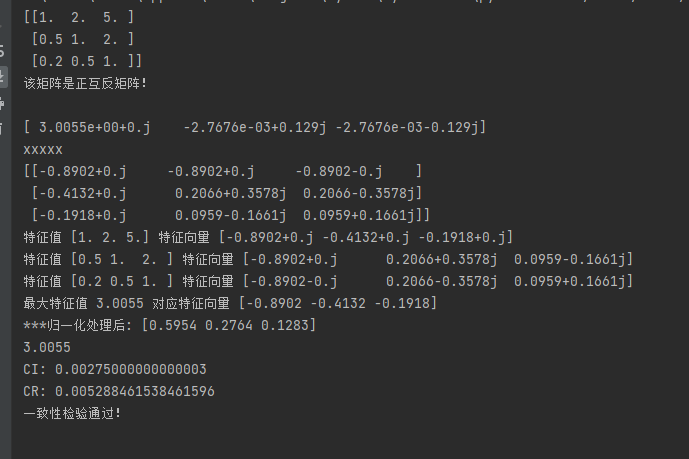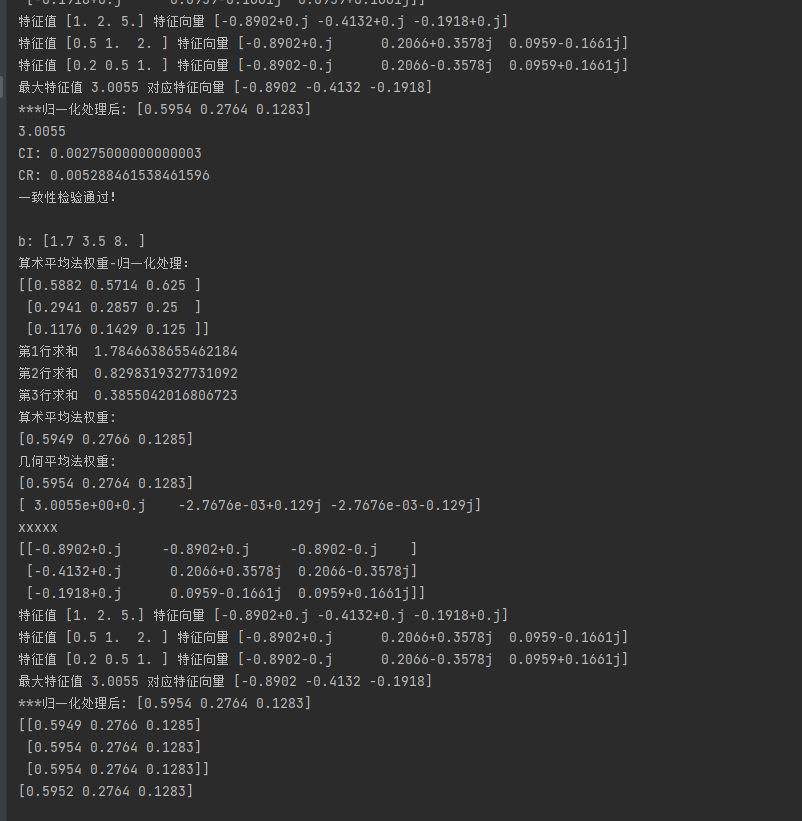# 对代码的小小的展望：

这里笔者使用的print()函数较多，运行CI_calc()CR_calc() 函数时候，都会调用eigenvalue()函数，就会重复打印其中的特征值和对应的特征向量，这里可以优化清风老师是使用特征值法求的权重，得出最终的权重矩阵（这里我就直接使用清风老师的内容了，不再去单独做综合三种方法得到的权重的excel）# ⑤填充矩阵，得出结果

苏杭得分：指标权重×苏杭与其他两种方案中的权重，即前两列相乘：
0.5954×0.2636＋0.0819×0.4758＋0.4286×0.0538＋0.6337×0.0981＋0.1667×0.1087=0.299

# AHP扩展知识

1、评价的决策层不能太多，太多的话n会很大，判断矩阵和一致矩阵差异可能会很大。因为平均随机一致性指标 RI 的表格中 n 最多是15，因此应该根据实际情况选择是否应用此方法。
2、如果决策层中指标的数据是已知的，那么层次分析法不容易将这些已知数据应用在其中。如拿上面的例题举例：如果已知景色 、花费、居住、饮食以及交通在三个旅游景点的一些数据，那么如何将这些数据转化为构造判断矩阵的依据，只能为其提供一定的文字说明，而不容易将数据应用到其中。
3、在实际建模中，判断矩阵的数值都是人为填的，具有一定的主观性存在，这时应该搜寻相应的数据让人信服，不能空口无凭。

# 个人拙见

①关于AHP层次分析法就讲到这里，希望大家能多看几遍，可能后续建模或者论文中会涉及到，就我个人认为，虽然是专家填的判断矩阵，也使用客观的一致性检验，该模型应该算是主观的一类评价类模型。

②AHP层次分析法涉及到线性代数的一些内容，而其他的评价类模型，如DEA数据包络方法，其还涉及到运筹学相关知识（对偶问题）。